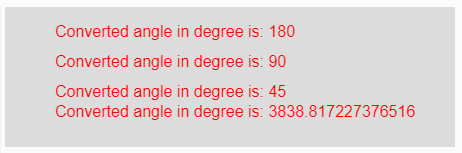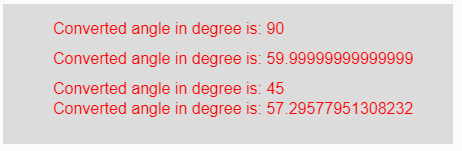GeeksforGeeks App
Open AppBrowser
Continue

# p5.js | degrees() function

The degrees() function in p5.js is used to convert a given radian measurement value to its corresponding value in degrees.

Syntax:

`degrees( radian )`

Parameters: This function accepts single parameter radian which is to be converted into degrees.

Return Value: It returns the converted angle into degrees.

Below programs illustrate the degrees() function in p5.js:

Example 1: This example uses degrees() function to convert given radian measurement value to its corresponding value in degrees.

 `function` `setup() { ``  ` `    ``// Creating Canvas size``    ``createCanvas(450, 140); ``} `` ` `function` `draw() { ``      ` `    ``// Set the background color ``    ``background(220); ``    ` `    ``// Initializing some angles in radians``    ``let Rad1 = PI; ``    ``let Rad2 = PI / 2; ``    ``let Rad3 = PI / 4; ``    ``let Rad4 = 67; ``     ` `     ` `    ``// Calling to degrees() function``    ``let A = degrees(Rad1);``    ``let B = degrees(Rad2);``    ``let C = degrees(Rad3);``    ``let D = degrees(Rad4);``     ` `    ``// Set the size of text ``    ``textSize(16); ``      ` `    ``// Set the text color ``    ``fill(color(``'red'``)); ``    ` `    ``// Getting converted angles into degree``    ``text(``"Converted angle in degree is: "` `+ A, 50, 30);``    ``text(``"Converted angle in degree is: "` `+ B, 50, 60);``    ``text(``"Converted angle in degree is: "` `+ C, 50, 90);``    ``text(``"Converted angle in degree is: "` `+ D, 50, 110);``} `

Output:Example 2: This example uses degrees() function to convert given radian measurement value to its corresponding value in degrees.

 `function` `setup() { ``  ` `    ``// Creating Canvas size``    ``createCanvas(450, 140); ``} `` ` `function` `draw() { ``      ` `    ``// Set the background color ``    ``background(220); ``     ` `    ``// Calling to degrees() function with different``    ``// radians value as parameter``    ``let A = degrees(PI/2);``    ``let B = degrees(PI/3);``    ``let C = degrees(PI/4);``    ``let D = degrees(PI/PI);``     ` `    ``// Set the size of text ``    ``textSize(16); ``      ` `    ``// Set the text color ``    ``fill(color(``'red'``)); ``    ` `    ``// Getting converted angles into degree``    ``text(``"Converted angle in degree is: "` `+ A, 50, 30);``    ``text(``"Converted angle in degree is: "` `+ B, 50, 60);``    ``text(``"Converted angle in degree is: "` `+ C, 50, 90);``    ``text(``"Converted angle in degree is: "` `+ D, 50, 110);``}  `

Output:Reference: https://p5js.org/reference/#/p5/degrees

My Personal Notes arrow_drop_up
Related Tutorials